NEET  >  31 Year NEET Previous Year Questions: Atoms

# 31 Year NEET Previous Year Questions: Atoms

Test Description

## 40 Questions MCQ Test Physics Class 12 | 31 Year NEET Previous Year Questions: Atoms

31 Year NEET Previous Year Questions: Atoms for NEET 2023 is part of Physics Class 12 preparation. The 31 Year NEET Previous Year Questions: Atoms questions and answers have been prepared according to the NEET exam syllabus.The 31 Year NEET Previous Year Questions: Atoms MCQs are made for NEET 2023 Exam. Find important definitions, questions, notes, meanings, examples, exercises, MCQs and online tests for 31 Year NEET Previous Year Questions: Atoms below.
Solutions of 31 Year NEET Previous Year Questions: Atoms questions in English are available as part of our Physics Class 12 for NEET & 31 Year NEET Previous Year Questions: Atoms solutions in Hindi for Physics Class 12 course. Download more important topics, notes, lectures and mock test series for NEET Exam by signing up for free. Attempt 31 Year NEET Previous Year Questions: Atoms | 40 questions in 80 minutes | Mock test for NEET preparation | Free important questions MCQ to study Physics Class 12 for NEET Exam | Download free PDF with solutions
 1 Crore+ students have signed up on EduRev. Have you?
31 Year NEET Previous Year Questions: Atoms - Question 1

### What is the radius of iodine atom (At. no. 53,mass no. 126)          

Detailed Solution for 31 Year NEET Previous Year Questions: Atoms - Question 1

53 electrons in iodine atom are distributed as
2, 8, 18, 18, 7
∴ n = 5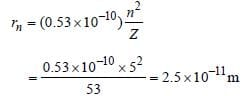31 Year NEET Previous Year Questions: Atoms - Question 2

### The ionisation energy of hydrogen atom is 13.6eV, the ionisation energy of helium atom wouldbe     

Detailed Solution for 31 Year NEET Previous Year Questions: Atoms - Question 2

E ∝ Z2 and Z for helium = 2
∴ (E)He  = 4x13.6 = 54.4 eV

31 Year NEET Previous Year Questions: Atoms - Question 3

### To explain his theory, Bohr used 

Detailed Solution for 31 Year NEET Previous Year Questions: Atoms - Question 3

Bohr used conservation of angular
momentum.

31 Year NEET Previous Year Questions: Atoms - Question 4

The ground state energy of H-atom 13.6 eV. Theenergy needed to ionize H-atom from its secondexcited state. 

Detailed Solution for 31 Year NEET Previous Year Questions: Atoms - Question 4

Second excited state corresponds to n = 3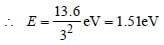31 Year NEET Previous Year Questions: Atoms - Question 5

In terms of Bohr radius a0, the radius of the second Bohr orbit of a hydrogen atom is given by       

Detailed Solution for 31 Year NEET Previous Year Questions: Atoms - Question 5

As r ∝ n2 , therefore, radius of 2nd
Bohr’s orbit = 4 a0.

31 Year NEET Previous Year Questions: Atoms - Question 6

The ionization energy of hydrogen atom is 13.6eV. Following Bohr’s theory, the energycorresponding to a transition between 3rd and4th orbit is 

Detailed Solution for 31 Year NEET Previous Year Questions: Atoms - Question 6

According to Bohr's theory,
En​= −13.6​/ n2
∴E3​=−13.6​/32=−13.6/9​=−1.51eV
E4​=−13.6/42​=−13.6/16​=−0.85eV
Energy corresponding to a transition between the 3rd and 4th orbit =E4​−E3​=−0.85−(−1.51) =0.66eV
Hence, the energy corresponding to a transition between the 3rd and 4th orbit is 0.66eV.

31 Year NEET Previous Year Questions: Atoms - Question 7

Which source is associated with a line emissionspectrum ? 

Detailed Solution for 31 Year NEET Previous Year Questions: Atoms - Question 7

Neon street sign is a source of line emission
spectrum.

31 Year NEET Previous Year Questions: Atoms - Question 8

In Rutherford scattering experiment, what willbe the correct angle for α-scattering for an impactparameter, b = 0 ? 

Detailed Solution for 31 Year NEET Previous Year Questions: Atoms - Question 8

Impact parameter for Rutherford scattering
experiment,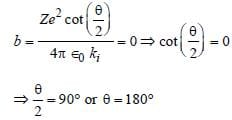31 Year NEET Previous Year Questions: Atoms - Question 9

The radius of hydrogen atom in its ground stateis 5.3 × 10–11 m. After collision with an electron itis found to have a radius of 21.2 × 10–11 m. Whatis the principal quantum number n of the finalstate of the atom           

Detailed Solution for 31 Year NEET Previous Year Questions: Atoms - Question 9

r ∝ n2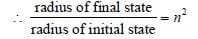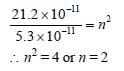31 Year NEET Previous Year Questions: Atoms - Question 10

The spectrum obtained from a sodium vapourlamp is an example of 

Detailed Solution for 31 Year NEET Previous Year Questions: Atoms - Question 10

A spectrum is observed, when light coming
directly from a source is examined with a
spectroscope. Therefore spectrum obtained
from a sodium vapour lamp is emission
spectrum.

31 Year NEET Previous Year Questions: Atoms - Question 11

When a hydrogen atom is raised from the groundstate to an excited state, 

Detailed Solution for 31 Year NEET Previous Year Questions: Atoms - Question 11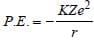and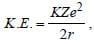where,
r is the radius of orbit which increases as we
move from ground to an excited state.
Therefore, when a hydrogen atom is raised
from the ground state, it increases the value
of r. As a result of this, P.E. increases
(decreases in negative) and K.E. decreases.

31 Year NEET Previous Year Questions: Atoms - Question 12

If the threshold wavelength for a certain metal is 2000 Å, then the work-function of the metal is           

Detailed Solution for 31 Year NEET Previous Year Questions: Atoms - Question 12

Threshold wavelength (λ) = 2000 Å = 2000 ×
10–10 m. Work function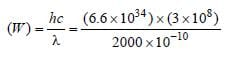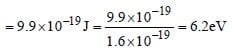31 Year NEET Previous Year Questions: Atoms - Question 13

When hydrogen atom is in its first excited level,its radius is      

Detailed Solution for 31 Year NEET Previous Year Questions: Atoms - Question 13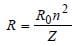Radius in ground state =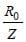Radius in first excited state =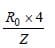(∵ n =2)
Hence, radius of first excited state is four
times the radius in ground state.

31 Year NEET Previous Year Questions: Atoms - Question 14

In the Bohr model of a hydrogen atom, the centripetal force is furnished by the coulomb attraction between the proton and the electron. If a0 is the radius of the ground state orbit, m is the mass, e is the charge on the electron and ε0 is the vacuum  ermittivity, the speed of the electron is                 

Detailed Solution for 31 Year NEET Previous Year Questions: Atoms - Question 14

Centripetal force = Coulombian force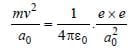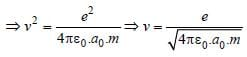31 Year NEET Previous Year Questions: Atoms - Question 15

Who indirectly determined the mass of theelectron by measuring the charge of the electron?     

Detailed Solution for 31 Year NEET Previous Year Questions: Atoms - Question 15

Explanation : The mass of the electron is discovered by  the Millikan by the oil drop experiment.

E = mg

=> qE = mg

q/m = g/E

Therefore, charge was quantised.

31 Year NEET Previous Year Questions: Atoms - Question 16

When an electron jumps from the fourth orbit tothe second orbit, one gets the      

Detailed Solution for 31 Year NEET Previous Year Questions: Atoms - Question 16

When the electron drops from any orbit to
second orbit, then wavelength of line
obtained belongs to Balmer series.

31 Year NEET Previous Year Questions: Atoms - Question 17

Which of the following transitions in a hydrogenatom emits the photon of highest frequency?         

Detailed Solution for 31 Year NEET Previous Year Questions: Atoms - Question 17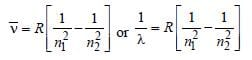Frequency,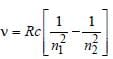Note : See the greatest energy difference and
also see that the transition is from higher to
lower energy level. Hence, it is highest in
case of n = 2 to n = 1.

31 Year NEET Previous Year Questions: Atoms - Question 18

An electron changes its position from orbit n = 2 to the orbit n = 4 of an atom. The wavelength of the emitted radiations is (R = Rydberg’s constant)

Detailed Solution for 31 Year NEET Previous Year Questions: Atoms - Question 18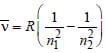, where n1 = 2, n2 = 4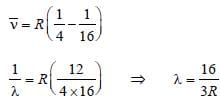31 Year NEET Previous Year Questions: Atoms - Question 19

The energy of hydrogen atom in nth orbit is En, then the energy in nth orbit of single ionised helium atom will be 

Detailed Solution for 31 Year NEET Previous Year Questions: Atoms - Question 19

We have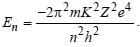For helium
Z = 2. Hence requisite answer is 4En.

31 Year NEET Previous Year Questions: Atoms - Question 20

J.J. Thomson’s cathode-ray tube experimentdemonstrated that  

Detailed Solution for 31 Year NEET Previous Year Questions: Atoms - Question 20

Cathode rays are streams of negatively
charged ions

31 Year NEET Previous Year Questions: Atoms - Question 21

In which of the following systems will the radiusof the first orbit (n = 1) be minimum ? 

Detailed Solution for 31 Year NEET Previous Year Questions: Atoms - Question 21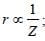Z(=3) is maximum for Li2+.

31 Year NEET Previous Year Questions: Atoms - Question 22

The Bohr model of atoms   

Detailed Solution for 31 Year NEET Previous Year Questions: Atoms - Question 22

In Bohr’s model, angular momentum is
quantised i.e  =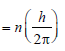31 Year NEET Previous Year Questions: Atoms - Question 23

Energy E of a hydrogen atom with principal quantum number n is given by E = – 13.6/n2 eV. The energy of photon ejected when the electronjumps from n = 3 state to n = 2 state of hydrogenis approximately 

Detailed Solution for 31 Year NEET Previous Year Questions: Atoms - Question 23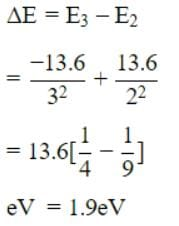31 Year NEET Previous Year Questions: Atoms - Question 24

The half life of radium is about 1600 years. Of 100 g of radium existing now, 25 g will remainunchanged after 

Detailed Solution for 31 Year NEET Previous Year Questions: Atoms - Question 24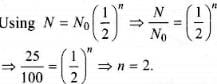The total time in which radium change to 25 g is

= 2 x 1600

= 3200 yr.

31 Year NEET Previous Year Questions: Atoms - Question 25

The total energy of an electron in the firstexcited state of hydrogen atom is about –3.4eV. Its kinetic energy in this state is 

Detailed Solution for 31 Year NEET Previous Year Questions: Atoms - Question 25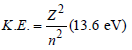Mechanical energy =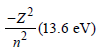∴ K.E. in 2nd orbital for hydrogen
= – Mechanical energy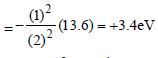31 Year NEET Previous Year Questions: Atoms - Question 26

Ionization potential of hydrogen atom is 13.6eV.Hydrogen atoms in the ground state are excitedby monochromatic radiation of photon energy12.1 eV. According to Bohr’s theory, the spectrallines emitted by hydrogen will be 

Detailed Solution for 31 Year NEET Previous Year Questions: Atoms - Question 26

Energy of ground state 13.6 eV
Energy of first excited state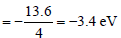Energy of second excited state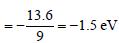Difference between ground state and 2nd
excited state = 13.6 – 1.5 = 12.1 eV
So, electron can be excited upto 3rd orbit
No. of possible transition
1 → 2, 1 → 3, 2 → 3
So, three lines are possible

31 Year NEET Previous Year Questions: Atoms - Question 27

The total energy of electron in the ground stateof hydrogen atom is – 13.6 eV. The kinetic energyof an electron in the first excited state is        

Detailed Solution for 31 Year NEET Previous Year Questions: Atoms - Question 27

Energy in the first excited state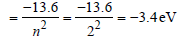But K.E. = –(Total energy) = +3.4 eV.

31 Year NEET Previous Year Questions: Atoms - Question 28

The ground state energy of hydrogen atom is 13.6eV. When its electron is in the first excited state, its excitation energy is 

Detailed Solution for 31 Year NEET Previous Year Questions: Atoms - Question 28

When the electron is in first excited state
(n = 2), the excitation energy is given by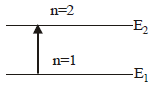ΔE = E2 – E1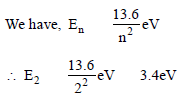Given E1 = –13.6eV
∴Δ E = ( – 3.4) – ( – 13.6) = 10.2 eV.

31 Year NEET Previous Year Questions: Atoms - Question 29

In a Rutherford scattering experiment when a projectile of charge Z1 and mass M1 approaches a target nucleus of charge Z2 and mass M2, the distance of closest approach is r0. The energy of the projectile is            

Detailed Solution for 31 Year NEET Previous Year Questions: Atoms - Question 29

The kinetic energy of the projectile is given by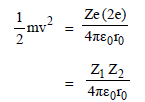Thus energy of the projectile is directly
proportional to Z1, Z2

31 Year NEET Previous Year Questions: Atoms - Question 30

The ionization energy of the electron in thehydrogen atom in its ground state is 13.6 eV.The atoms are excited to higher energy levels toemit radiations of 6 wavelengths. Maximumwavelength of emitted radiation corresponds tothe transition between 

Detailed Solution for 31 Year NEET Previous Year Questions: Atoms - Question 30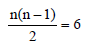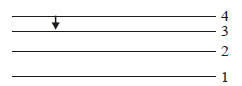n2 – n – 12 = 0
(n – 4) (n + 3) = 0 or n = 4

31 Year NEET Previous Year Questions: Atoms - Question 31

The energy of a hydrogen atom in the groundstate is – 13.6 eV. The energy of a He+ ion in thefirst excited state will be 

Detailed Solution for 31 Year NEET Previous Year Questions: Atoms - Question 31

Energy of a H-like atom in it's nth state is
given by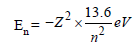For, first excited state of He+, n = 2, Z = 2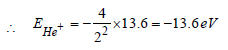31 Year NEET Previous Year Questions: Atoms - Question 32

An alpha nucleus of energy 1/2mv2  bombards a heavy nuclear target of charge Ze. Then the distance of closest approach for the alpha nucleus will be proportional to 

Detailed Solution for 31 Year NEET Previous Year Questions: Atoms - Question 32

Kinetic energy of alpha nucleus is equall to
electrostatic potential energy of the system
of the alpha particle and the heavy nucleus.
That is,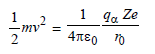where r0 is the distance of closest approach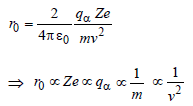Hence, correct option is (c).

31 Year NEET Previous Year Questions: Atoms - Question 33

The wavelength of the first line of Lyman seriesfor hydrogen atom is equal to that of the secondline of Balmer series for a hydrogen like ion. Theatomic number Z of hydrogen like ion is 

Detailed Solution for 31 Year NEET Previous Year Questions: Atoms - Question 33

For first line of Lyman series of hydrogen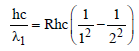For second line of Balmer series of hydrogen
like ion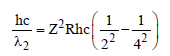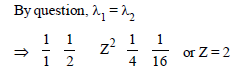31 Year NEET Previous Year Questions: Atoms - Question 34

An electron in the hydrogen atom jumps from excited state n to the ground state. The wavelength so emitted illuminates a
photosensitive material having work function 2.75 eV. If the stopping potential of thephotoelectron is 10 V, the value of n is [2011 M]

Detailed Solution for 31 Year NEET Previous Year Questions: Atoms - Question 34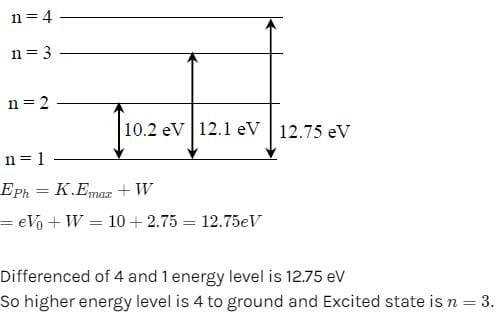31 Year NEET Previous Year Questions: Atoms - Question 35

Out of the following which one is not a possibleenergy for a photon to be emitted by hydrogenatom according to Bohr’s atomic model? [2011M]

Detailed Solution for 31 Year NEET Previous Year Questions: Atoms - Question 35

Obviously, difference of 11.1eV is not
possible.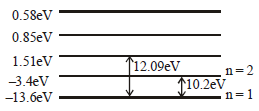31 Year NEET Previous Year Questions: Atoms - Question 36

Electron in hydrogen atom first jumps from third excited state to second excited state and then from second excited to the first excited state.The ratio of the wavelength λ1 : λ2 emitted inthe two cases is 

Detailed Solution for 31 Year NEET Previous Year Questions: Atoms - Question 36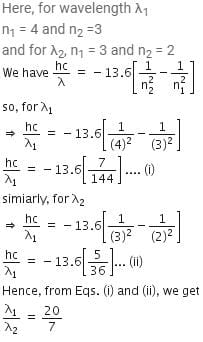31 Year NEET Previous Year Questions: Atoms - Question 37

An electron of a stationary hydrogen atom passes from the fifth energy level to the ground level. The velocity that the atom acquired as a result of photon emission will be : 

(m is the mass of the electron, R, Rydberg constant and h Planck’s constant)

Detailed Solution for 31 Year NEET Previous Year Questions: Atoms - Question 37

For emission, the wave number of the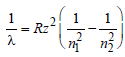R= Rydberg constant, Z = atomic number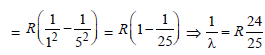linear momentum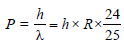(de-Broglie hypothesis)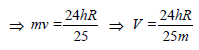31 Year NEET Previous Year Questions: Atoms - Question 38

The transition from the state n = 3 to n = 1 in ahydrogen like atom results in ultravioletradiation. Infrared radiation will be obtained inthe transition from : [2012M]

Detailed Solution for 31 Year NEET Previous Year Questions: Atoms - Question 38

The frequency of the transitionwhen n = 1, 2, 3.

31 Year NEET Previous Year Questions: Atoms - Question 39

The ratio of longest wavelengths corresponding to Lyman and Blamer series in hydrogen spectrum is [NEET 2013]

Detailed Solution for 31 Year NEET Previous Year Questions: Atoms - Question 39

For Lyman series (2 → 1)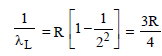For Balmer series (3 → 2)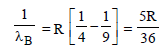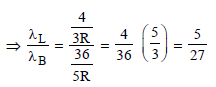31 Year NEET Previous Year Questions: Atoms - Question 40

An electron in hydrogen atom makes a transition n1 → n2 where n1 and n2 are principal quantum numbers of the two states. Assuming Bohr’s model to be valid the time period of the electron in the initial state is eight times that in the final state. The possible values of n1 and n2 are        [NEET Kar. 2013]

Detailed Solution for 31 Year NEET Previous Year Questions: Atoms - Question 40

T ∝ n3
Tn1 = 8 Tn2 (given)
Hence, n1 = 2n2

## Physics Class 12

157 videos|452 docs|213 tests
 Use Code STAYHOME200 and get INR 200 additional OFF Use Coupon Code
Information about 31 Year NEET Previous Year Questions: Atoms Page
In this test you can find the Exam questions for 31 Year NEET Previous Year Questions: Atoms solved & explained in the simplest way possible. Besides giving Questions and answers for 31 Year NEET Previous Year Questions: Atoms, EduRev gives you an ample number of Online tests for practice

## Physics Class 12

157 videos|452 docs|213 tests

### How to Prepare for NEET

Read our guide to prepare for NEET which is created by Toppers & the best Teachers